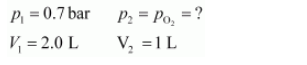# What will be the pressure of the gaseous mixture when 0.5 L of H2 at 0.8 bar and

Question:

What will be the pressure of the gaseous mixture when 0.5 L of H2 at 0.8 bar and 2.0 L of dioxygen at 0.7 bar are introduced in a 1L vessel at 27°C?

Solution:

Let the partial pressure of H2 in the vessel be.

Now,

$\begin{array}{ll}p_{1}=0.8 \text { bar } & p_{2}=p_{H_{2}}=? \\ V_{1}=0.5 \mathrm{~L} & V_{2}=1 \mathrm{~L}\end{array}$

It is known that,

$p_{1} V_{1}=p_{2} V_{2}$

$\Rightarrow p_{2}=\frac{p_{1} V_{1}}{V_{2}}$

$\Rightarrow p_{H_{2}}=\frac{0.8 \times 0.5}{1}$

$=0.4 \mathrm{bar}$

Now, let the partial pressure of $\mathrm{O}_{2}$ in the vessel be $p_{\mathrm{O}_{2}}$.

Now,$\mathrm{p}_{1} \mathrm{~V}_{1}=\mathrm{p}_{2} \mathrm{~V}_{2}$

$\Rightarrow \mathrm{p}_{2}=\frac{\mathrm{p}_{1} \mathrm{v}_{1}}{\mathrm{v}_{2}}$

$\Rightarrow \mathrm{PO}_{2}=\frac{0.7 \times 2.0}{1}=1.4 \mathrm{bar}$

Total pressure of the gas mixture in the vessel can be obtained as:

$p_{\text {total }}=p_{\mathrm{H}_{2}}+p_{\mathrm{O}_{2}}$

$=0.4+1.4$

$=1.8$ bar

Hence, the total pressure of the gaseous mixture in the vessel is $1.8$ bar .